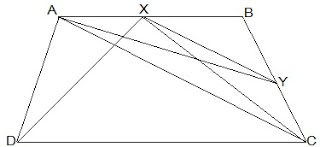Guru

# ABCD is a trapezium with AB || DC. A line parallel to AC intersects AB at X and BC at Y. Prove that ar (△ADX) = ar (△ACY). [Hint : Join CX.] Q/13

• 0

What is the best way for solving the question from class 9th ncert math of Areas of Parallelograms and Triangles chapter of Ncert of exercise 9.3of math. What is the best way for solving this question please guide me the best way for solving this question. ABCD is a trapezium with AB || DC. A line parallel to AC intersects AB at X and BC at Y. Prove that ar (△ADX) = ar (△ACY). [Hint : Join CX.]

Share

1.Given,

ABCD is a trapezium with AB || DC.

XY || AC

Construction,

Join CX

To Prove,

Proof:

ar(△ADX) = ar(△AXC) — (i) (Since they are on the same base AX and in-between the same parallels AB and CD)

also,

ar(△ AXC)=ar(△ ACY) — (ii) (Since they are on the same base AC and in-between the same parallels XY and AC.)

(i) and (ii),